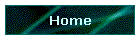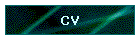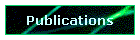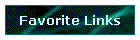#### آذر, 1383### Papers in English

1. The Strong Law of Large Numbers for N.D Generalized Gaussian R.V ,Stochastic.Anal.Appl,(2000).

2. Negative Dependent Bounded R.V Probability Inequalities and S.L.L.N,Appl.Math.Stochastics.Anal,(2000),(with M.Amini).

3. The Almost Some Convergence of Weighted Sums of N.D ,R.V Journal of Science ,(1999),( With M.Amini and H.Azarnush).

4. The Strong Law of Large Numbers and Asymptotic Behavior of Quantiles for Negative R.V ,Math and Computer in Science and Engineering ,(2001).

5. Chung Type Strong Laws for Arrays of Random Elements and Bootstrapping ,Stochastic .Anal.Appl,(1997),(with  Patterson,R.F, and Taylor,R.L).

6. Weak Laws of Large Numbers for Arrays of Rowwise N.D Random Variables,Acta See Math,(2001).

7. A Strong Law of Large Number for Arrays of R.V Rowwise N.D,Stochastic.Anal.Appl.(2001).

8. Linex Bayes Estimation of Weibull Distribution ,Adv.Appl.Stat,(2001),(With Gandomi and Noorbaloochi).

9. Weak Laws of Large Numbers for N.D R.V in Banach Spaces ,Research Development in Probability and Statistics,(1996),(with Patterson,R.F and Taylor,R.L).

10. A Strong Law for Arrays of i.i.d Random Elements in a Separable Balance Space [Banach Space],26th Annual Iranian Mathematics Conference,(1995).

11. Moving Average and Complete Convergence ,Iranian Math,(1994),(with Sadeghi).

12. On the Laws of Large Numbers for Rowwise Conditionaly Independent Random elements , Internat. Journal of Math. Math. Sci. (1993), (with  Patterson R.F and Taylor,R.L).

13. Some Comments on a Result of Han\vs on Strong Convergence of Sequences of Random Elements in Separable Banach spaces ,Math.Slovaca,(1989),(With Bhaskara ,Rao.M).

14. Measurability and Convergence Theorems for Random Elements in Banach Spaces in Terms of Total Subsets of Linear Functionals ,Bull.Inst.Math.Acad.Sinica.,(1986),(With Bhaskara,Rao.M).

15.  A Note on Some Characterizations of Various Type of Convergence- Bull.Inst.Math.Acad.Sinica,(1986),(With Bahaskara,Rao.M).

16. On Weakly Uncorrelated Random Elements ,9th National Mathematics Conference,(1979).

17. On an Extention of Some Result of Chow and Lai on Limit Theorems for Weighted Sums of Independent Random Variables to Separable Banach Spaces,Bull.Inst.Math.Acad.Sinica,(1981),(With Bhaskara,Rao.M).

18. Limit Theorems for Weighted Sums of Random Elements in Separable Banach Spaces,J.Multivariate Anal,(1979),(With Bhaskara,Rao.M).

19. On Weak Orthogonality and a Strong Law of Large Numbers ,Bull. Acad.Polon.Sci.Sci.Math.Astronom.Phys.(1978),(With Bhaskara,Rao.M).

20. A Weak Law of Large Numbers for Sequences of Pairwise Independent Identicaly Distributed Random Elements,8th National Mathematics Conference,(1977).

21. Some Remarks on the Strong Law of Large Numbers for Banach-Space Valued weakly Integrable Random Variables,J.Multivariate.Anal.(1978),(With Bahaskara,Rao.M).

22. ON Summability Methods and Limits Theorems for Banacha Space Valued Random Variables,Bull.Inst.Math.Acad.Sinica,(1979),(With Bahaskara,Rao.M).

23. A Strong Law of Large Numbers for Subsequence of Random Elements in Separable Banach Spaces,Ann.Probab,(1979),(With Bahaskara,Rao.M).

24. A Strong Law of Large Number for N.D Variables, Internat.Appl.Math.Conference,(1998),(With Amini).

25. A Weak And Strong Convergence for Subgaussian Variables,Appl.Math.Confernce,(2001),(with Amini).

26. Convergence in Probability for Sequence of R.V in Separable Banach Space,9th National Math Conference,(1979).

27. From Crisp Random Variable to Fazian Random Variable,Vo.1,(2002),(With M.Amini).

28. Limit Theorems for Dependent R.V ,Proceeding of the First World Congress of Nonlinear Analysis,(1996).

29. Chung S.L.L.N's for Array of V.E and Bootstrapping,Mulivariate Analysis,.Proceeding of240-256,(1996).

30. Strong Laws of Large Numbers for Arrays of Conditionally Independent R.E ,J.Appl.Matha& Statistic Analysis.

31. Negation Dependent and Large Law of Large Numbers,   Proceeding of 25th Annual I.M.S ,(1996).

32. Measuribility and Convergence in Probability,Proceeding of 13th Applied Math,(1997).

33. Probability Intequalities for N.D Bounded ,R.V Applied Math ,Proceeding of 118,(1999).

34. A Strong Law of Large Numbers,proceeding of 26th Annual I.M.C,?????,??????.

35. The S.L.L.N for Negatively Dependent to Appear ,in Journal of Stochastic Analysis.

36. An Almost Sure Convergence Theorems for R.V,30th Iranian Internat.Conference ,(1999).

37. Some Strong Limit Theorem for N.D, R.V,The Second Iranian Seminar On Probability and Stochastic Process,(1999).

38. Laws of Large Numbers for N.D,R.V, The Second Iranian Seminar on Stochastic Process,?????,??????,???????.

39. Limit Theorems for Dependent R.V.S, First Congress of Nonlinear Analysis,(1996),???.

40. The Strong Law of Large Number for Pairwise Negatively Dependent Random Variable,Second WSEAS Multiconference on Applied and Theoretical  Mathematics ,Carins ,Australia,(2001).

41. Sub-Gaussian Techniques in Proving Some Strong Limit Theorems, Second WSEAS Multiconference on Applied and Theoretical Mathematics,Carins,Australia,(2001).

42. Probability Inequalitiy for ND R.V.S, Statistcs,Operational Research II, Second WSEAS Multiconference on Applied and Theoretical  Mathematics,Carins,Austalia,(2001).

43. Convergence of Weighted Sums of Random Variables,Second WSEAS Multiconference on Applied and Theoretical Mathematics,Carins,Australia,(2001) 17-93 .

44. Limits Theorems for  Negatively Dependent Random Variables, Nonlinear Analysis,(2001).

45. On the Limit Theorems for Arrays of Rowwise PND R.V.S, Journal of inst of Math & Comp.Scv. Vol 16 No 1,(2003) 5-12.

46.  On the L.L.N for Dependent R.V.S , Journal of Sci, Islamic Republic of Iran, (2003).

### Books in Farsi

1. Time Series Analysis and  Forecasting, Translate.

2. Basics of Sampling, Translate, (With Sadeghi).

3. Sampling ,Translate, (With Hosseinion).

4. Data Concise and Error Analysis, Translate, (With Edalai).

5. Multivariate Statistical Inference ,Translate.

6. Time Series and Forecasting ,Authored, (With Niromand).

7. Control Theory ,Translate, (With Vahidian Kamyad).

8. Deferential Equations and Stationarity Theory, Translate, (With Hendi Khezrbeygi).

9. Solution Manual for Mathematical Analysis Vol 1,Authored .

10. Solution Manual for Mathematical Analysis Vol 2,Authored.

11. Probability Models Application ,Translate.

12. Statistics Theory Vol1,Translate.

13. Statistics Theory Vol2,Translate.

15. Basics of Mathematical Analysis from Topological View Point, Translate, (With Niknam).

16. Basics of Mathematical Physics, Translate ,(With Edalati).

17. Frequency Analysis of events and Risks , Translate.

18. Basics of Arithmetics ,Translate, (With Niknam).

19. Applied Multivariate Statistics ,Translate ,(With Arghami).

20. Design and Analysis of Agricultural Experiments,Translate,(With Saraphraz).

21. Categorical Statistical Models,Translate ,(With Meshkani).

22. Family of Gamma Distribution , Translate,(With Rezai Pajand).

23. An Introduction to Time Series Analysis, Translate, (With Niromand).

24. Numerical Algorithms, Translate ,(With Totonian Mashhad).

25. Statistics and Probability in Engineering ,Translate ,(With Assadi).

26. Modern Probability Theory ,Translate, (With Ala).

27. Design of Experiments ,Authored, (With Shahkar).

28. A First Course in Probability, Translate, (With Meshkani and Niromand and Azarnush).

29. Quality Control, Authored, (With Tareghian).

30. Applied Statistics ,Translate ,(With Tehranian).

31. Engineering Mathematics ,Translate ,(With Keraye Chian).

32. Calculus ,Translate, (With Vahidian Kamyad).

33. Basics of Probability , Translate , (With Shahkar ).

34. A First Course in Probability , Translate,(With Iranmanesh).

35. How to Solve it by Computer? ,Translate,(With Gheysari Gholami).

36. Regression Analysis ,Translate , (With Shahkar).

37. Stochastics Processes, Translate, (With Shahkar).

38. Elements to Statistics and Probability ,??????,?????.

39. Survival Analysis , Translate, (With Rezai Pajand).

40. An Introduction to Sampling ,Translate , (With Arghami and Sanjari).

41. Limit  Theorems for Negatively Dependent Random Variable and Random Elements, ?????, (With Patterson,R.F and Taylor ,R.l).

Home | CV | Publications | Favorite Links

This site was last updated 09/26/83